Categories

# Light into the light Java Sorting Algorithm

Java String sorting algorithm source code

I. Introduction

Q: What is the choice?
Selection is assumed that a set number N, to determine the maximum value by which the K th. For example, A and B which is larger objects need? Another example: to consider to find the maximum number of items from an array?

Select solve the problem, we need to have a target capacity, that is to compare any two objects, and determine which large, which is equal to or smaller. Find solutions to the biggest problem of the item, followed by relatively long object (Comparable) capacity, circulation object list, one will be able to solve.

So how JDK source code to achieve more (Comparable) the ability of it?

Two, java.lang.Comparable Interface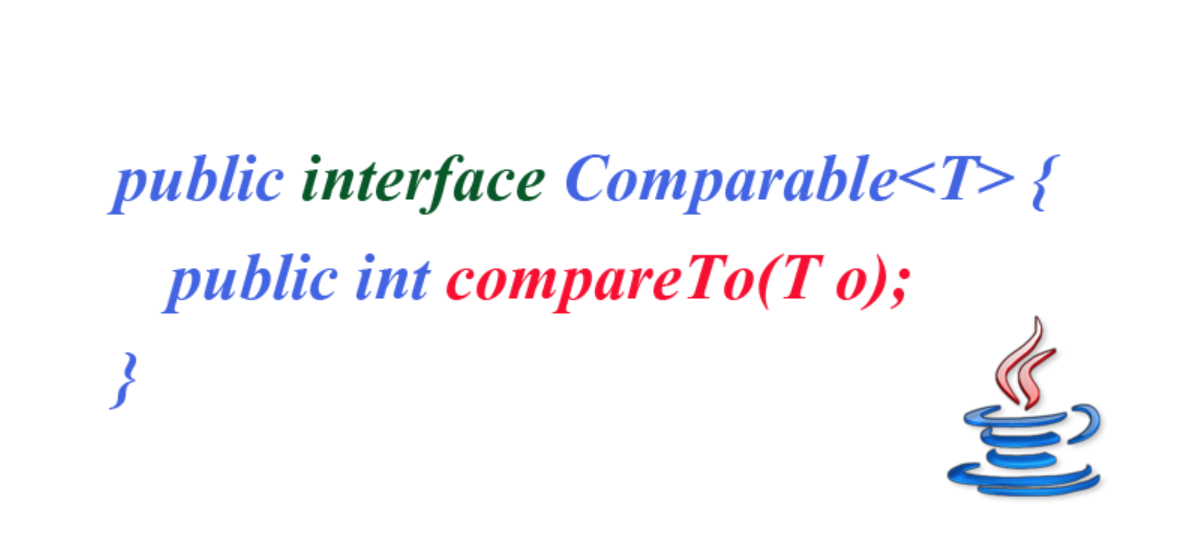Comparable interface, there will be a version of JDK 1.2, considered a long history. Comparable interface enforces sorted list of objects implementation class. Which is called the natural order sort, which compareTo method, known as the natural comparison.

The interface has a method public int compareTo (T o);, it can be seen

Into the reference T o: implement the interface class corresponding to the incoming object to be compared

Return Value int: positive, negative, and 0, representing greater than, less than and equal to

Set list (Collection List) or an array of objects (arrays), but also the corresponding tools can easily use:

java.util.Collections # sort (List) List sorting

java.util.Arrays # sort (Object []) sorted array

How that String object to be compared?

Three, String algorithm source code

String source String JDK 1.0 can be seen there. So when should JDK 1.2, String class implements the Comparable interface, and the need to pass the object being compared is String. Objects Figure: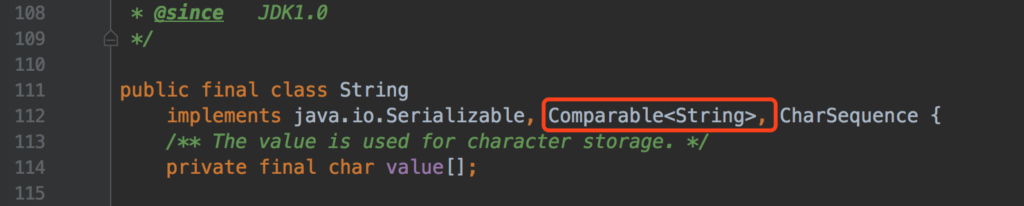String is a final class can not extend a new class from the String. From line 114, it can be seen that the storage structure is a character string (Char) array. First you can look at a string comparison case, the code is as follows:

``````
/**
* 字符串比较案例
*
* Created by bysocket on 19/5/10.
*/
public class StringComparisonDemo {

public static void main(String[] args) {
String foo = "ABC";

// 前面和后面每个字符完全一样，返回 0
String bar01 = "ABC";
System.out.println(foo.compareTo(bar01));

// 前面每个字符完全一样，返回：后面就是字符串长度差
String bar02 = "ABCD";
String bar03 = "ABCDE";
System.out.println(foo.compareTo(bar02)); // -1 (前面相等,foo 长度小 1)
System.out.println(foo.compareTo(bar03)); // -2 (前面相等,foo 长度小 2)

// 前面每个字符不完全一样，返回：出现不一样的字符 ASCII 差
String bar04 = "ABD";
String bar05 = "aABCD";
System.out.println(foo.compareTo(bar04)); // -1  (foo 的 'C' 字符 ASCII 码值为 67，bar04 的 'D' 字符 ASCII 码值为 68。返回 67 - 68 = -1)
System.out.println(foo.compareTo(bar05)); // -32 (foo 的 'A' 字符 ASCII 码值为 65，bar04 的 'a' 字符 ASCII 码值为 97。返回 65 - 97 = -32)

String bysocket01 = "泥瓦匠";
String bysocket02 = "瓦匠";
System.out.println(bysocket01.compareTo(bysocket02));// -2049 （泥 和 瓦的 Unicode 差值）
}
}
``````

Results are as follows:

``````0
-1
-2
-1
-32
-2049
``````

As can be seen, compareTo method is to compare two strings lexicographically. Comparison of specific rules can look at the code comments. Compare the following rules:

Each character string is exactly the same, returns 0

Each character of the string is exactly the same as the previous section, the return: the length difference is behind two strings

• 字符串前面部分的每个字符存在不一样，返回：出现不一样的字符 ASCII 码的差值

Comparative Chinese Unicode code value corresponding to the return (Unicode contains ASCII)

foo ‘C’ in the character ASCII code is 67

bar04 ‘D’ in the character ASCII code is 68.

foo.compareTo (bar04), returns 67–68 = -1

Common ASCII character code, as shown in Figure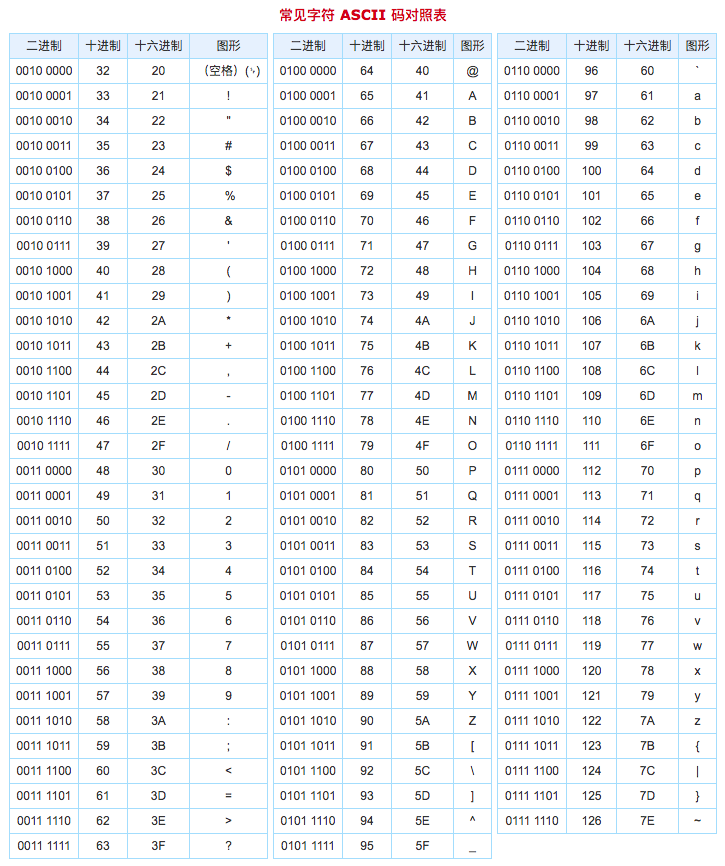Look at how the String compareTo method to achieve dictionary order. Source Figure: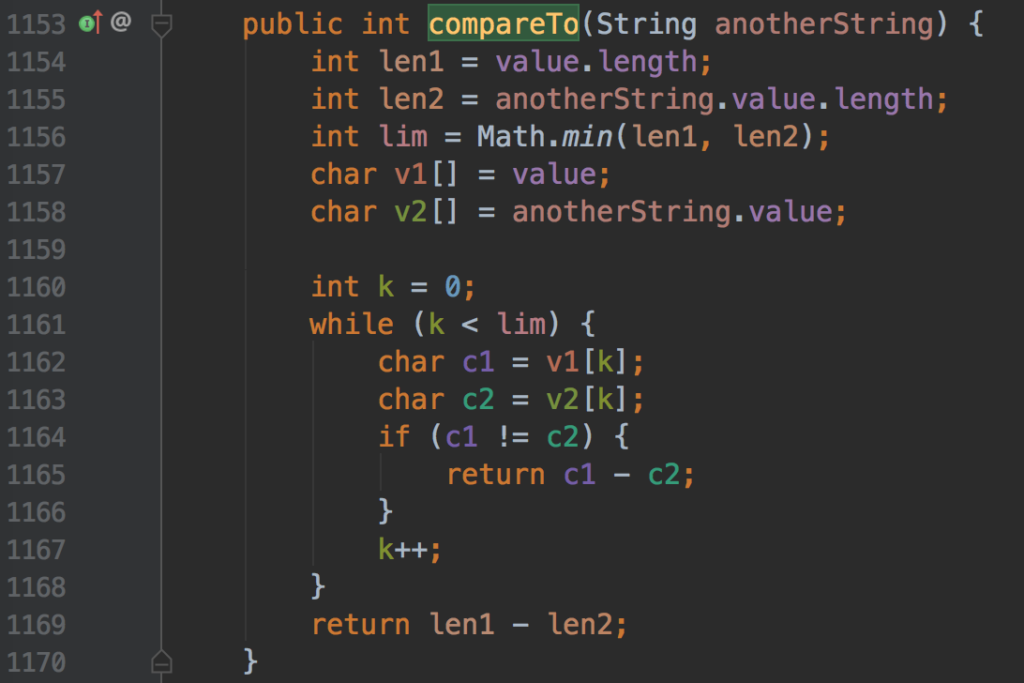Source interpreted as follows:

Line 1156: Get the current string and another string, a smaller length value lim

Line 1161: if lim is greater than 0 (a small non-empty string), the comparison is started

1164 line: the current string and another string, followed by comparing character. If the difference is not equal, returns two characters of Unicode encoding value

1169 line: the current string and another string, followed by comparing character. If both are equal, the difference between the length of the two strings is returned

So to sort, be sure to have more capability, that implement the Comparable interface. Then achieve this object list (and arrays) may be ordered via the interface the Collections.sort (and Arrays.sort).

There TreeSet implemented using a tree structure (red-black tree), sort the elements of the collection. Which sort it is to achieve this interface Comparable

In addition, if not implement the Comparable interface, using the sort, java.lang.ClassCastException will throw an exception. The details look at “Java collections: Three, HashSet, TreeSet and compare LinkedHashSet” https://www.bysocket.com/archives/195

IV Summary

The above also said, in fact, this comparison has some drawbacks:

CompareTo not ignore the default character case. If you want to ignore, then re-customize compareTo method

Decisions can not be compared in two dimensions. For example, to determine 2 * 3 * 3 1 rectangle and rectangle, which is bigger?

For example, some classes can not implement the interface. A final class can not extend a new class. It also has the solution: a function object (Function Object)

Method Parameters: define a class not only process the data, and pass an instance of the class. A function by placing it inside a target to be transferred. Such objects often called function object (Funtion Object)

Interface design method, T execute callback using similar (the Callback callback) parameter. Spring example, in source code, many designs can be seen that: in the polymerization priority inheritance or implemented. This can reduce a lot of inherited or achieved. Similar SpringJdbcTemplate scene design, consider the design and implementation of such a Callback.

That insertion sort it?

Article Project:

• JDK 1.8
• Project name: algorithm-core-learning

I. Introduction

The above sorting algorithm Java String source, speaking what is the choice, what is more capacity.

Selection is assumed that a set number N, to determine the maximum value by which the K th. Algorithm for solving a problem.

What is an algorithm?
It is a collection of some algorithm, a simple set of instructions, a simple set of instructions are specified. The algorithm to determine the important indicators:

The first if that resolves the problem;

The second algorithm running time, that is, to solve the problem of how much time the results required;

There needed space resources, such as memory.

In many cases, not enough to write a work program. Because encounter large data, run time is an important issue.

Algorithm performance is represented by a large O notation. O mark notation is large relative growth rate, accuracy is rough. For example 2N and 3N + 2, it is O (N). It is often said of linear growth, there is often said that the exponential growth, etc.

Typical growth rates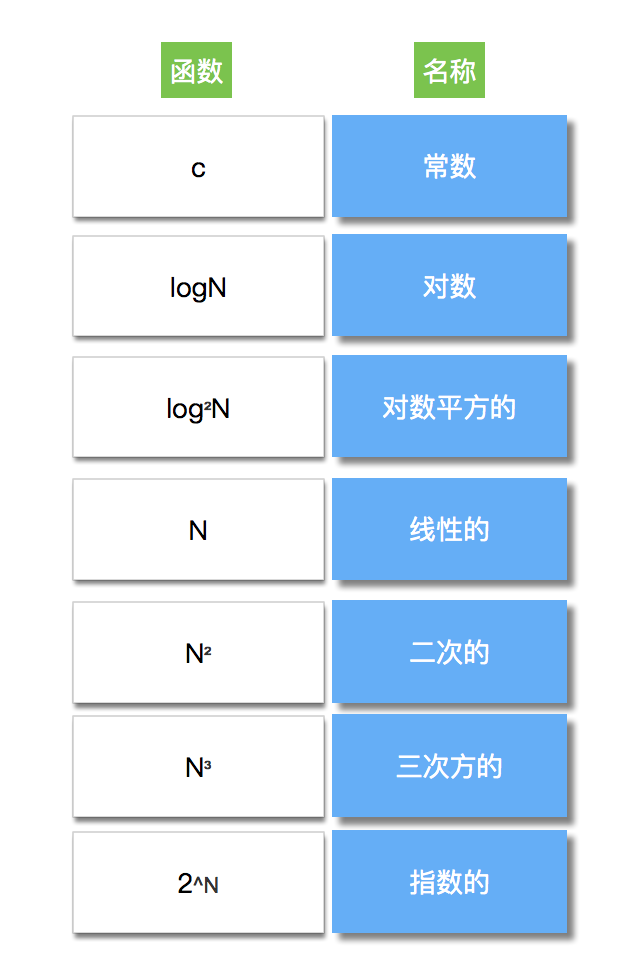A typical approach is to provide the performance of divide and conquer, that branch of divide and conquer strategy:

The problem is divided into two approximately equal sub-problems recursively solving them, which is a sub-portion;

The two sub-stages of treatment solution to the problem of patching together, and a small amount of additional work to do again, finally got the solution of the whole problem.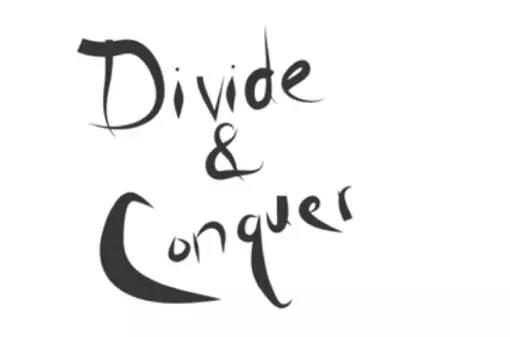Second, sorting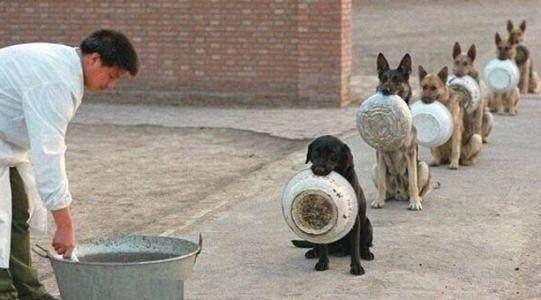Scheduling problem, is old, but has been a popular question. ACM from exposure to work now, each involving algorithms, some algorithms or JDK source code reading materials, there is often a sorting algorithm appears.

Sorting algorithm to a set of arrays (or sequence) rearranging the data arrangement in line with the descending (or ascending) order. Such data from disorder to order, what benefits?

• 应用层面：解决问题。

The easiest is to find the maximum or minimum

Solve the “together” of the problem, ie, the same mark elements together

Match two or more files in the project

By keycode find information

• System level: to reduce the entropy of the system, increasing the degree of order system
(Donald Knuth’s classic “The Art of Computer Programming” (The Art of Computer Programming) Volume III)

Done in the main memory is called sorting, internal order. That needs to complete disk and other storage sorting, called external sorting external sorting. Profile Address: https: //en.wikipedia.org/wiki/External_sorting

Previous “Java String sorting algorithm source code”, talked about java.lang.Comparable interface. So interface is an abstract type, is a set of abstract method (the compareTo), and with the interface declared. Therefore the Comparable sorting objects belonging to type, i.e. realized Comparable interface, and then call an object method compareTo achieved compared sorted.

Under these conditions the sorting, called sorted based on the comparison (comparison-based sorting)

Third, insertion sort

Vernacular: Big Bear (a), bear two, three … bear in accordance with height from low to high queue (sorted). This time the bear N join the team, it started to compare teams from the tail. If it is lower than the height of the front bear, the comparison with the exchange positions, sequentially comparing & exchange position from the tail to the head. The final change to the location where N should bear. This is the principle of insertion sort.

Insertion sort (insertion sort)

One of the simplest sort. ps: look like bubble sort is not recommended for learning

• 由 N – 1 次排序过程组成。

If such an element is sorted, you do not need sorting. I.e., N = 1 (1 – 1 = 0)

Each sort assurance, from the first position to the current position state elements are sorted.

• Figure: compare each element forward, and terminate at their location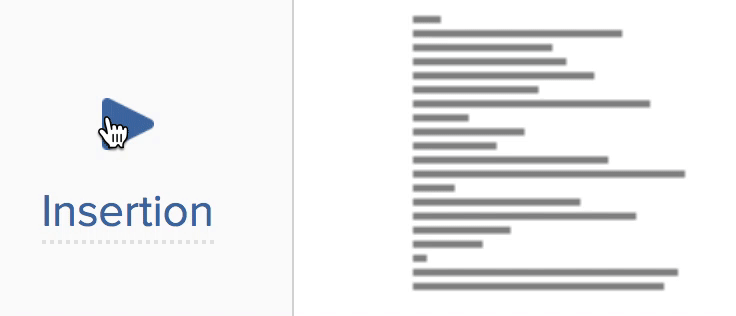``````/**
* 插入排序案例
* ``````
* Created by 泥瓦匠@bysocket.com on 19/5/15.
*/
public class InsertionSortingDemo {

/**
* 插入排序
*
* @param arr 能比较的对象数组
* @param  已排序的对象数组
*/
public static  void insertionSort(T[] arr) {
int j;

// 从数组第二个元素开始，向前比较
for (int p = 1; p < arr.length; p++) {
T tmp = arr[p];
// 循环，向前依次比较
// 如果比前面元素小，交换位置
for (j = p; (j > 0) && (tmp.compareTo(arr[j - 1]) < 0); j--) {
arr[j] = arr[j - 1];
}
// 如果比前面元素大或者相等，那么这就是元素的位置，交换
arr[j] = tmp;
}
}

public static void main(String[] args) {
Integer[] intArr = new Integer[] {2, 3, 1, 4, 3};

System.out.println(Arrays.toString(intArr));
insertionSort(intArr);
System.out.println(Arrays.toString(intArr));
}
}``````

Code analysis as follows:

From the second element of the array, start comparing forward. Smaller than the first element, then the switch position

If the second element of the comparison, then the third, fourth and so on ...

When comparing to the last element to complete the sequencing

Time complexity is O (N ^ 2), the best scenario is that the sorted had been properly arranged, that is O (N), because it can not satisfy the condition determination cycle; most extreme array in reverse order, that is O ( N ^ 2). Therefore, the time complexity of the algorithm is O (N ^ 2)

Running the main method, the following results:

``````[2, 3, 1, 4, 3]
[1, 2, 3, 3, 4]``````

Then think about optimization, will be how to optimize it?
Insertion Sort optimized version than the more forward. Comparison of the forward half, two points more likely to be better. Specific code, can themselves try

Four insertion sort, Array.sort source of

The above insertion algorithm used to sort themselves to achieve, in fact Array.sort JDK provides a method for easy sorting. Case code is as follows:

``````/**
* Arrays.sort 排序案例
* ``````
* Created by 泥瓦匠@bysocket.com on 19/5/28.
*/
public class ArraysSortDemo {

public static void main(String[] args) {

Integer[] intArr = new Integer[] {2, 3, 1, 4, 3};

System.out.println(Arrays.toString(intArr));
Arrays.sort(intArr);
System.out.println(Arrays.toString(intArr));
}
}``````

Running the main method, the following results:

``````[2, 3, 1, 4, 3]
[1, 2, 3, 3, 4]``````

That Arrays.sort is how to achieve it? With JDK 1.2 when Arrays, JDK 1.8 version when optimizing a sort algorithm. As follows:

If the number of elements is less than 47, using an insertion sort

If the number of elements is less than 286, using a fast sort

Timsort integration algorithm merge sort and insertion sort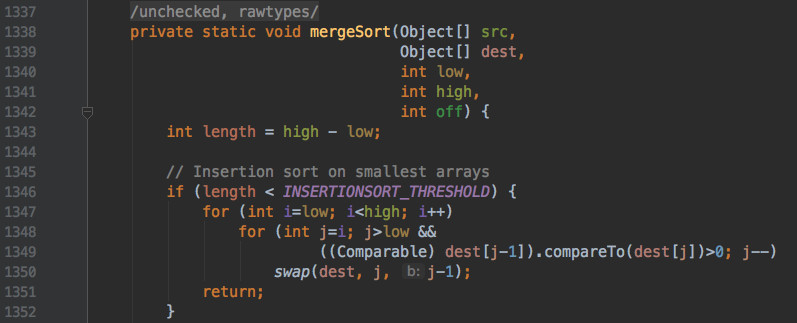Source code we see mergeSort which integrates the insertion sort algorithm to achieve the same purpose with the above. Here, not line by line explanation.

V. Summary

Algorithm to solve the problem. It is not necessarily an algorithm to solve a problem that may solve a problem with more than one. To achieve the optimal solution. Insertion sort, so it's that simple

The sample code

Readers can view the examples in this article by the following warehouse: StringComparisonDemo string comparison Case Case:

• Github：https://github.com/JeffLi1993/algorithm-core-learning
• Gitee：https://gitee.com/jeff1993/algorithm-core-learning

Reference material

"Data Structures and Algorithms analysis: Java language to describe the (original book 3rd edition)"

• https://en.wikipedia.org/wiki/Unicode
• https://www.cnblogs.com/vamei/tag/%E7%AE%97%E6%B3%95/
• https://www.bysocket.com/archives/2314/algorithm